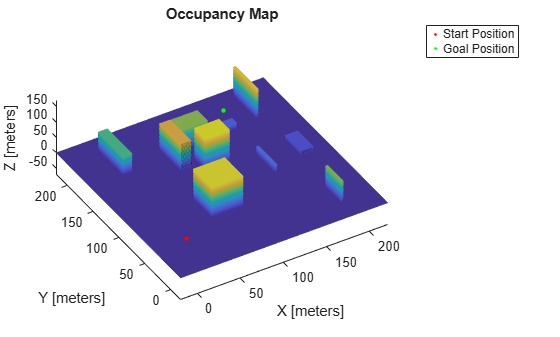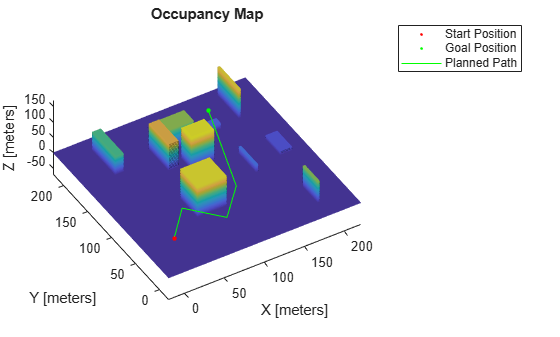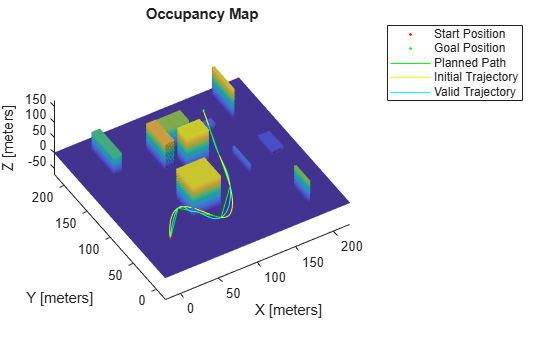Plan Minimum Snap Trajectory for Quadrotor

This example shows how to plan a minimum snap trajectory (minimum control effort) for a multirotor unmanned aerial vehicle (UAV) from a start pose to a goal pose on a 3D map by using the optimized rapidly exploring random tree (RRT*) path planner.

In this example, you set up a 3D map, provide a start pose and goal pose, plan a path with RRT* using 3D straight line connections, and fit a minimum snap trajectory through the obtained waypoints.

Initial Setup

Configure the random number generator for repeatable result.

rng(100,"twister")

Load the 3D occupancy map uavMapCityBlock.mat, which contains a set of pregenerated obstacles, into the workspace. The occupancy map is in an east-north-up (ENU) frame.

omap = mapData.omap;

% Consider unknown spaces to be unoccupied
omap.FreeThreshold = omap.OccupiedThreshold;
show(omap)

Set Start Pose and Goal Pose

Using the map for reference, select an unoccupied start pose and goal pose for the trajectory.

startPose = [12 22 25 0.7 0.2 0 0.1];  % [x y z qw qx qy qz]
goalPose = [150 180 35 0.3 0 0.1 0.6];

% Plot the start and goal poses
hold on
scatter3(startPose(1),startPose(2),startPose(3),100,".r")
scatter3(goalPose(1),goalPose(2),goalPose(3),100,".g")
view([-31 63])
legend("","Start Position","Goal Position")
hold offPlan Path with RRT* Using SE(3) State Space

RRT* is a tree-based motion planner that builds a search tree incrementally from random samples of a given state space. The tree eventually spans the search space and connects the start state and the goal state. Connect the two states with straight line connections using a stateSpaceSE3 object. Use the validatorOccupancyMap3D object for collision checking between the multirotor UAV and the environment.

Define State Space Object

The stateSpaceSE3 object defines the state space as [x y z qw qx qy qz], where [x y z] specifies the position of the UAV in meters and [qw qx qy qz] specifies the orientation as a quaternion. Specify the position and orientation boundaries of the quadrotor as a 7-by-2 matrix. The orientation boundaries are optional, and can be set to -Inf and Inf if they are not applicable.

ss = stateSpaceSE3([-20 220;
-20 220;
-10 100;
inf inf;
inf inf;
inf inf;
inf inf]);

Define State Validator Object

The validatorOccupancyMap3D object determines that a state is invalid if the xyz-location is occupied on the map. A motion between two states is valid only if all intermediate states are valid, which means the UAV does not pass through any occupied locations on the map. Create a validatorOccupancyMap3D object by specifying the state space object and the inflated map. Then set the validation distance, in meters, for interpolating between states.

sv = validatorOccupancyMap3D(ss,Map=omap);
sv.ValidationDistance = 0.1;

Set Up RRT* Path Planner

Create a plannerRRTStar object by specifying the state space and state validator as inputs. Set the MaxConnectionDistance, GoalBias, MaxIterations, ContinueAfterGoalReached, and MaxNumTreeNodes properties of the planner object to optimize the returned waypoints.

planner = plannerRRTStar(ss,sv);
planner.MaxConnectionDistance = 50;
planner.GoalBias = 0.8;
planner.MaxIterations = 1000;
planner.ContinueAfterGoalReached = true;
planner.MaxNumTreeNodes = 10000;

Execute Path Planning

Perform RRT* based path planning in 3D space to obtain waypoints. The planner finds a flight path that is collision-free and suitable for the quadrotor. The solution info is helpful for tuning the planner.

[pthObj,solnInfo] = plan(planner,startPose,goalPose);

Visualize Path

Check if the RRT* planner has found a path. If the planner has found a path, plot the waypoints. Otherwise, terminate the script.

if (~solnInfo.IsPathFound)
disp("No Path Found by the RRT, terminating example")
return
end

% Plot map, start pose, and goal pose
show(omap)
hold on
scatter3(startPose(1),startPose(2),startPose(3),100,".r")
scatter3(goalPose(1),goalPose(2),goalPose(3),100,".g")

% Plot path computed by path planner
plot3(pthObj.States(:,1),pthObj.States(:,2),pthObj.States(:,3),"-g")
view([-31 63])
legend("","Start Position","Goal Postion","Planned Path")
hold offGenerate Minimum Snap UAV Trajectory

The original planned path has some sharp corners while navigating toward the goal. Generate a smooth trajectory by fitting the obtained waypoints to the minimum snap trajectory using the minsnappolytraj function. For your initial estimate of the time required to reach each waypoint, assume the UAV moves at a constant speed.

To tune the trajectory and flight time, specify the numSamples, TimeAllocation, and TimeWeight arguments of the minsnappolytraj function.

waypoints = pthObj.States;
nWayPoints = pthObj.NumStates;

% Calculate the distance between waypoints
distance = zeros(1,nWayPoints);
for i = 2:nWayPoints
distance(i) = norm(waypoints(i,1:3) - waypoints(i-1,1:3));
end

% Assume a UAV speed of 3 m/s and calculate time taken to reach each waypoint
UAVspeed = 3;
timepoints = cumsum(distance/UAVspeed);
nSamples = 100;

% Compute states along the trajectory
initialStates = minsnappolytraj(waypoints',timepoints,nSamples,MinSegmentTime=4,MaxSegmentTime=20,TimeAllocation=true,TimeWeight=100)';

Visualize Trajectory

Visualize the obtained minimum snap trajectory.

% Plot map, start pose, and goal pose
show(omap)
hold on
scatter3(startPose(1),startPose(2),startPose(3),30,".r")
scatter3(goalPose(1),goalPose(2),goalPose(3),30,".g")

% Plot the waypoints
plot3(pthObj.States(:,1),pthObj.States(:,2),pthObj.States(:,3),"-g")

% Plot the minimum snap trajectory
plot3(initialStates(:,1),initialStates(:,2),initialStates(:,3),"-y")
view([-31 63])
legend("","Start Position","Goal Postion","Planned Path","Initial Trajectory")
hold offGenerate Valid Minimum Snap Trajectory

Notice that the generated flight trajectory has some invalid states, which are not obstacle-free. You must modify the waypoints so that the generated trajectory is obstacle-free. To avoid invalid states, add intermediate waypoints on the segment between the pair of waypoints for which the trajectory is invalid. Insert waypoints iteratively until the entire trajectory is valid.

% Check if the trajectory is valid
states = initialStates;
valid = all(isStateValid(sv,states));

while(~valid)
% Check the validity of the states
validity = isStateValid(sv,states);

% Map the states to the corresponding waypoint segments
segmentIndices = exampleHelperMapStatesToPathSegments(waypoints,states);

% Get the segments for the invalid states
% Use unique, because multiple states in the same segment might be invalid
invalidSegments = unique(segmentIndices(~validity));

% Add intermediate waypoints on the invalid segments
for i = 1:size(invalidSegments)
segment = invalidSegments(i);

% Take the midpoint of the position to get the intermediate position
midpoint(1:3) = (waypoints(segment,1:3) + waypoints(segment+1,1:3))/2;

% Spherically interpolate the quaternions to get the intermediate quaternion
midpoint(4:7) = slerp(quaternion(waypoints(segment,4:7)),quaternion(waypoints(segment+1,4:7)),.5).compact;
waypoints = [waypoints(1:segment,:); midpoint; waypoints(segment+1:end,:)];

end

nWayPoints = size(waypoints,1);
distance = zeros(1,nWayPoints);
for i = 2:nWayPoints
distance(i) = norm(waypoints(i,1:3) - waypoints(i-1,1:3));
end

% Calculate the time taken to reach each waypoint
timepoints = cumsum(distance/UAVspeed);
nSamples = 100;
states = minsnappolytraj(waypoints',timepoints,nSamples,MinSegmentTime=2,MaxSegmentTime=20,TimeAllocation=true,TimeWeight=5000)';

% Check if the new trajectory is valid
valid = all(isStateValid(sv,states));

end

Visualize Final Valid Trajectory

Visualize the final valid minimum snap trajectory.

% Plot map, start and goal pose
show(omap)
hold on
scatter3(startPose(1),startPose(2),startPose(3),30,".r")
scatter3(goalPose(1),goalPose(2),goalPose(3),30,".g")

% Plot the waypoints
plot3(pthObj.States(:,1),pthObj.States(:,2),pthObj.States(:,3),"-g")

% Plot the initial trajectory
plot3(initialStates(:,1),initialStates(:,2),initialStates(:,3),"-y")

% Plot the final valid trajectory
plot3(states(:,1),states(:,2),states(:,3),"-c")
view([-31 63])
legend("","Start Position","Goal Postion","Planned Path","Initial Trajectory","Valid Trajectory")
hold offUAV Toolbox DocumentationGet trial now